We will now begin a 12-part series on the Platonic solids.  The study of the Platonic solids is the foundation for everything discussed in Cosmic Core.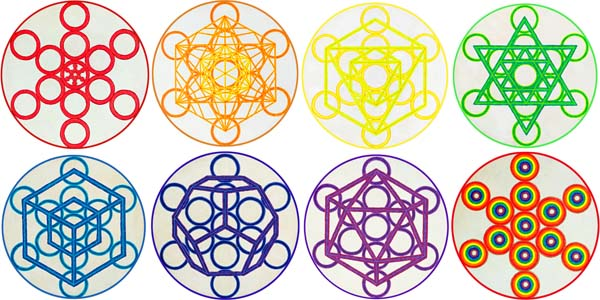# Mater into Matter

Consciousness forms into Light; Light into Geometric Form; Geometric Form into Matter

A sphere representing undifferentiated light stirs, polarizing into opposites symbolized by two intersecting circles (Vesica Piscis).  The two poles swirl in opposite directions in mathematically precise rhythmic energy patterns, creating a toroidal flow.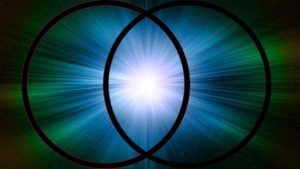The sphere becomes a whirling energy field (torus) displaying lines of force (geometry) upon which atoms configure to manifest material, visible volumes.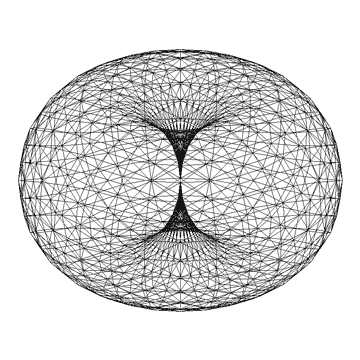This process above can be likened to the excitation of Aether Units.  These units are manifestations of fluctuations of Consciousness.

One photon may be the result of trillions of Aether units!

This information is discussed in detail in the Science section of Cosmic Core.  See Articles 98-129.

Photons rotate due to the rotating nature of the Aether itself.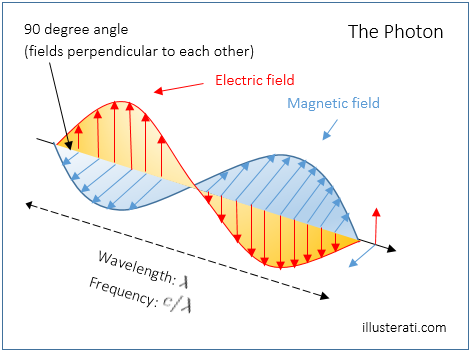Aether units are rotating spheres (toroids) with all geometry present in potentiation.  These units are not ‘things’.  They are an energy flow process.

Mass particles are composed of confined photons (vs. free photons).

Confined photons loop and spin in various ways to form subatomic particles.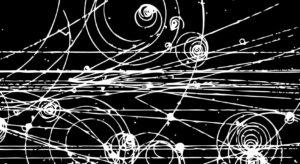The direction of looping (clockwise vs. anticlockwise) determines charge (positive or negative).

Charge simply results from the direction of spin of a particle formed from a photon.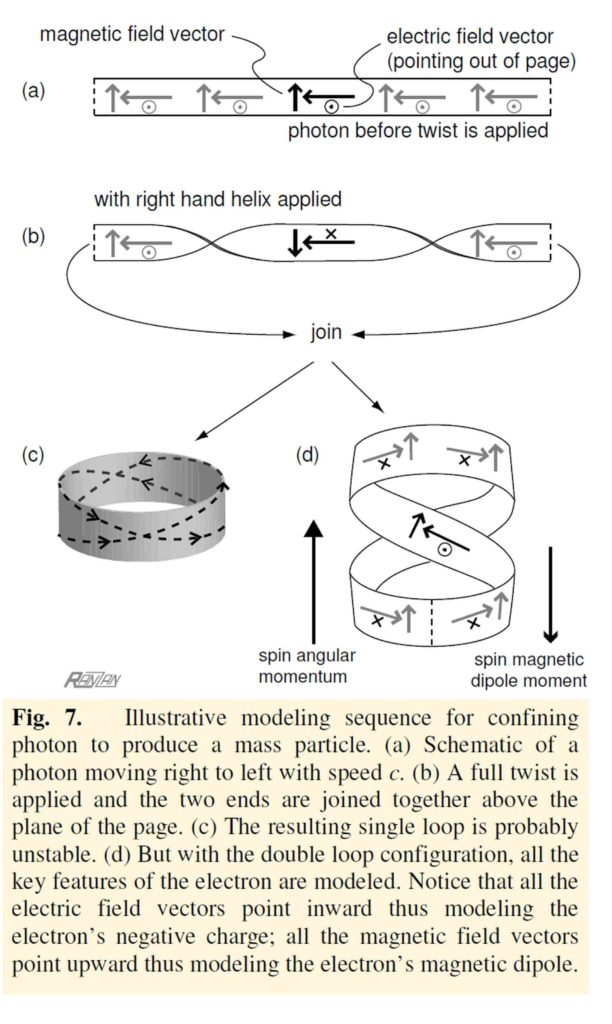As Conrad Ranzan writes, “The electron is a purely electromagnetic vortex.  With each wave cycle – with each resonance – the electron re-creates itself from itself.”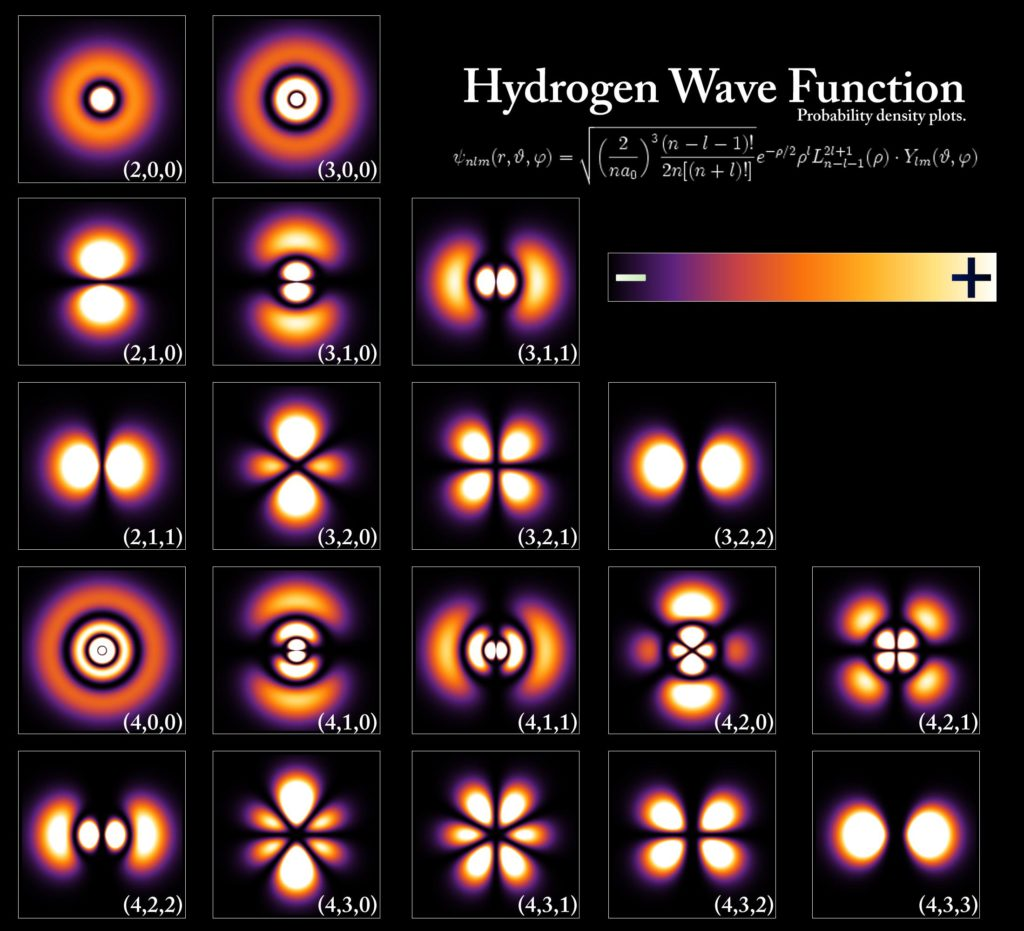Electron probability patterns of hydrogen.  Atoms are resonances.  That is, they are standing waves of Aether brought on by fluctuations of consciousness on a cosmic scale.

All particles are patterns (geometry) of excitation of the essence medium (Aether).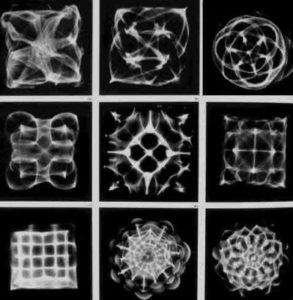Cymatics Patterns.  Proof that vibration (wavelength, frequency, harmonics) creates geometry.  This is a basic indisputable fact of existence.  ‘Particles’ are standing waves of geometry just like these Cymatics patterns.

Photon confinement is the essential role in all mass-particle formation.

Atoms are standing waves of rotating photons.

Chemical elements are specific rotational combinations of photons that have formed specific atoms with specific geometry.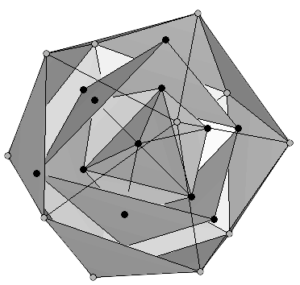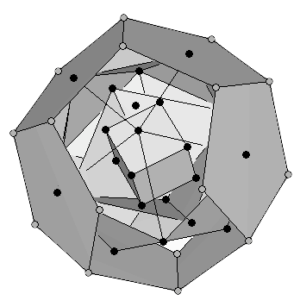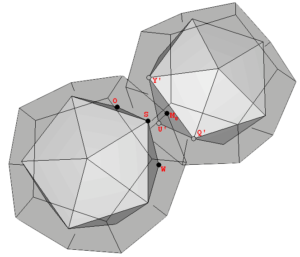An atom of Iron, Palladium and Uranium.  Credit:  Dr. Robert Moon  See Article 131

Photons confine and spin to create charged particles.  Spinning charged particles form atoms.  Atoms combine to form molecules.  Molecules combine to create matter.

And all these are formed upon geometric lines of force that determine their shape, structure and function.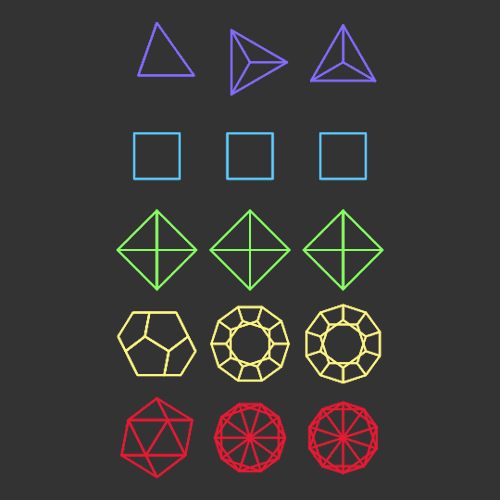These geometric lines of force are the Platonic solids and all combinations, truncations, and stellations.

All of this info is carefully laid out in the Science section in great detail.

# The Cosmic (Platonic) Solids

The five Platonic solids (and the sphere) are the most harmonic shapes in the universe.

They are the only five perfect three-dimensional forms (aside from the sphere).

They have more symmetry than any other shapes.

Their formal name is ‘regular polyhedra’.  They are also sometimes called ‘Cosmic solids’ or ‘Pythagorean solids’.

They are the:

• Tetrahedron
• Cube
• Octahedron
• Icosahedron
• Dodecahedron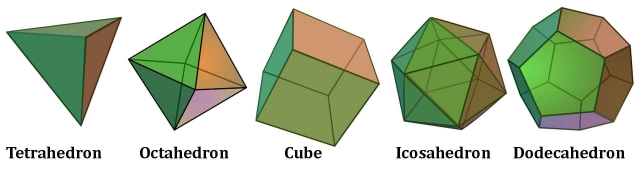They are the foundation or seeds of everything in the physical world.

# Known History of the Platonic Solids

Information about the Platonic solids has been taught since ancient times, particularly since the Greek Mystery Schools 2,500 years ago.

They are called “Platonic” after Plato (427 – 347 BC).In the Timaeus Plato outlines a cosmology through the metaphor of planar and solid geometry.

Because of this Plato was thought to have discovered these solids.

They were, however, known much earlier.

The Scotland stone sculptures were discovered at least 1000 years before Plato.

These “Scottish Solids” are 4,000 year old carved stone sets found at stone circles in Aberdeenshire, Scotland.Many, many solids were found.  See page 174 in Keith Critchlow’s Time Stands Still for a map of the sites.

They are being held in the Ashmolean Museum in Oxford, England.

The stones are carved into precise geometric spherical versions of each of the 5 solids, plus some compound and semi-regular solids.

Because of these features they can be called ’rounded squares’.

“They are a profound balance of understanding and expression between the measurable and immeasurable, that which is in time and the timeless, the changing and the changeless.”1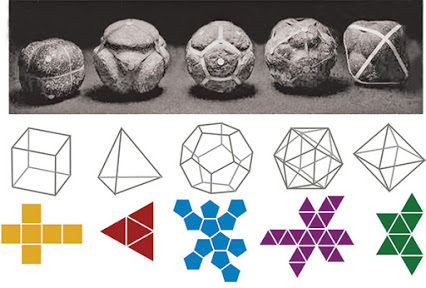The symbolism of the ‘rounded squares’ mirrors the symbolism of ‘Squaring the Circle’ that was discussed in Article 38.  The roundedness of the circle and the straight lines of the square represent a linking of cosmic consciousness with earth consciousness through the human consciousness.

Many of the stones are granite and would have taken months to complete – ‘permanence’ was of the essence.

The solids were found in a set of 9.  These represent the:

Tetrahedron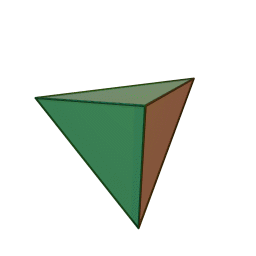Octahedron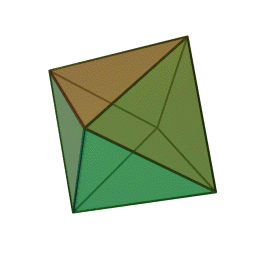Cube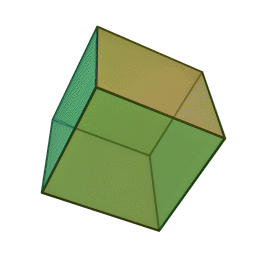Icosahedron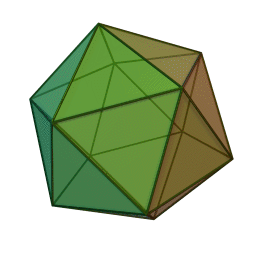Dodecahedron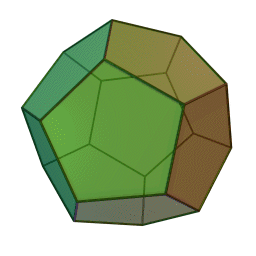Cuboctahedron (Archimedean solid) and its dual the rhombic dodecahedron (Catalan solid).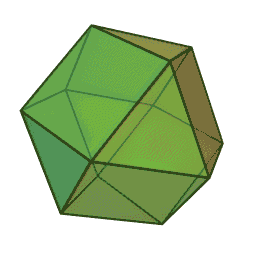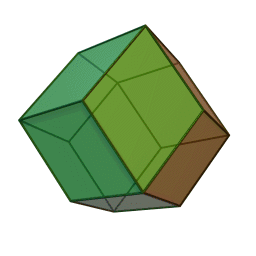Icosidodecahedron (Archimedean) and its dual the rhombic tricontahedron (Catalan)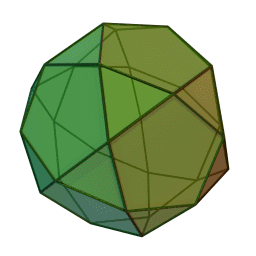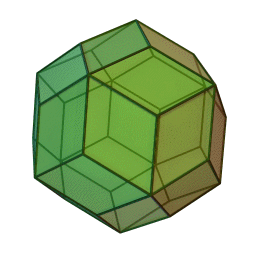Incidentally, when Critchlow examined the spheres in the Ashmolean, the curator, “had not deemed the spheres important enough to be on show but kept them in a drawer marked ‘Scotland’ with the other ‘lesser’ objects.”2

Other Platonic solid objects from ancient history were found elsewhere in the world.

These include:

Egyptian Icosahedral Dice with symbols carved into each face.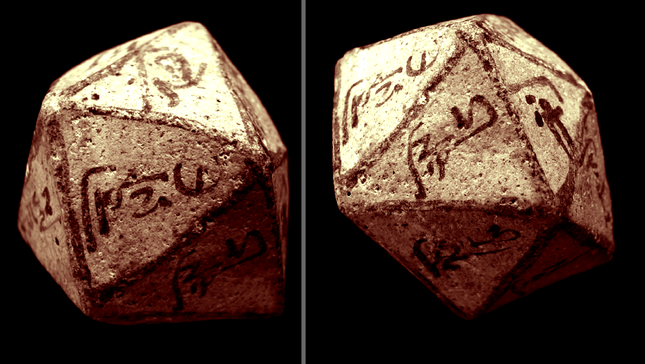Found from the Graeco-Roman period 1st century AD,  from the Dakhleh oasis. The original dice has a God’s name inscribed in Demotic (cursive Hieroglyphics) on each side.  Source

Greek Icosahedral Dice with Greek letters carved into each face.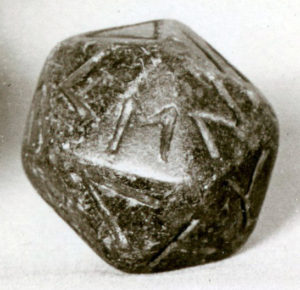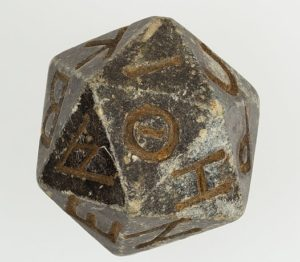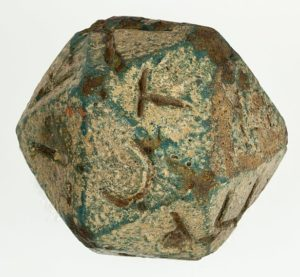2nd century B.C.–4th century A.D. – Ptolemaic Period–Roman Period.  These are currently housed in the MET in New York.

Etruscan dodecahedron with strange symbols carved in soapstone.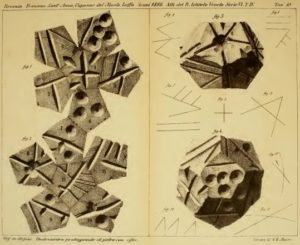This Neolithic dodecahedron made of ceramic was recovered at Monte Loffa, Italy. These markings, possibly from the Etruscan civilization, have never been deciphered.  Source

Etruscan dodecahedra found with little spheres on each point and curved iscosahdra.

The faces are not flat.  They curve to imply spherical geometry.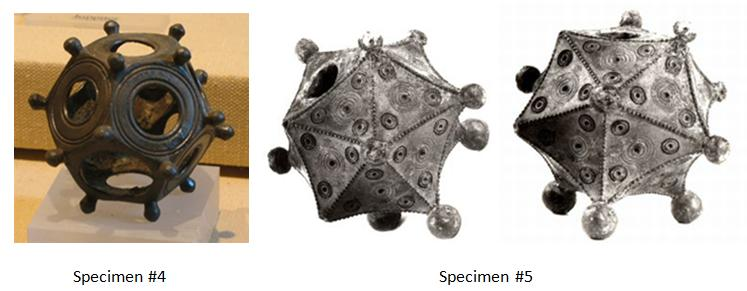Roman dodecahedra date from the 2nd or 3rd centuries CE.  About a hundred of these dodecahedra have been found from Wales to Hungary and to the east of Italy, with most found in Germany and France. Ranging from 4 cm to 11 cm in size, they also vary in terms of textures. Most of them are made of bronze but some also seem to be made of stone. Source

# Characteristics of the Platonic Solids

To review the previous article, the Platonic Solids are the only possible convex regular polyhedra.

A regular polygon has equal sides and equal lengths.

A regular polyhedron has equal regular polygon faces and identical vertices.

The five Platonic solids are the only shapes:

• with equal side lengths
• with equal interior angles
• that look the same from each vertex (corner point)
• with faces made of the same regular shape (triangle, square, pentagon) 3, 4, 5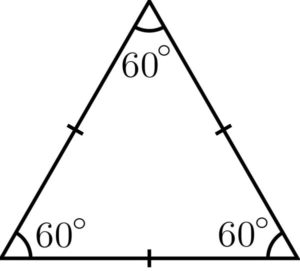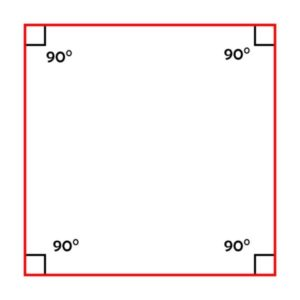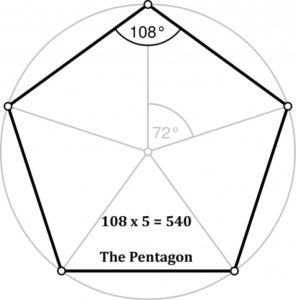• all fit perfectly in a sphere (circumsphere) with all points resting on the circumferenceAn example of this: the icosahedron

 Volume Face Shape Angle of Face Sum of Face Angles Sum of Volume Angles Reduces to 9 Tetrahedron Triangle 60 x 3 180 x 4 720 7 + 2 = 9 Octahedron Triangle 60 x 3 180 x 8 1440 1 + 4 + 4 = 9 Cube Square 90 x 4 360 x 6 2160 2 + 1 + 6 = 9 Icosahedron Triangle 60 x 3 180 x 20 3600 3 + 6 = 9 Dodecahedron Pentagon 108 x 5 540 x 12 6480 6 + 4 + 8 = 18 = 1 + 8 = 9

All Platonic Solids have a:

• Circumsphere – contains the solid; touches each vertex
• Midsphere (Intersphere) – passes through the midpoint of every edge
• Insphere – contained by the solid; perfectly touches the center of every face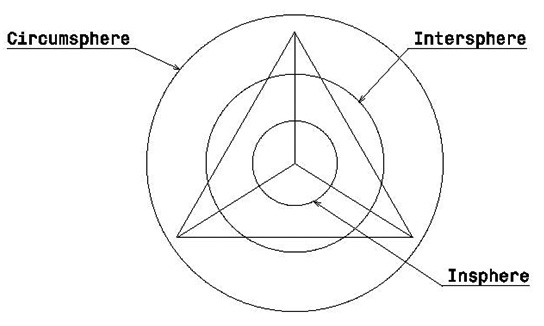All other regular volumes are truncations, combinations, rotations or stellations of these five.

As we see in Cymatics (Article 121), you can vibrate pure sound frequencies in water and get these shapes.  This is a key to understanding how matter is formed.  Invisible sound frequencies have a geometric structure.  Matter precipitates upon this structure.As discussed above, Aether units are the result of fluctuations of consciousness.  These fluctuations of consciousness create the invisible sound frequencies that contain the geometry upon which matter is formed.  In the case of consciousness, however, these ‘sound’ frequencies are beyond the range of our normal human hearing so we neither hear them nor see them yet they are present around us at all times.

All Platonic solids nest within each other in different ways.  This is discussed in detail in Article 44.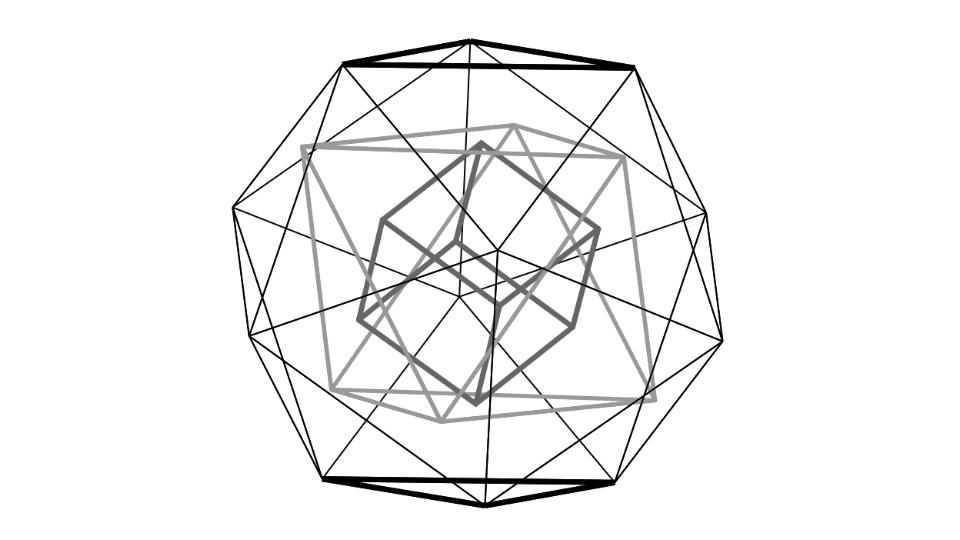In essence they are not five separate shapes, but five aspects of the same shape (the spinning sphere/torus).

When one Platonic solid is present, they are all present.  They cannot be separated.  They arise together as one – each in potentiation within the torus.  Certain shapes physically manifest depending on the nature of the manifestation (scale, function, type of life form…etc.).

Platonic solids are the core of how the universe is built from a living fluid-like universe.Furthermore, “The numbers involved in these volumes have been found to be identical to the spatial representations of musical harmonies.”3

# Geometry of Sound

The sum of the angles of each Platonic solid equals a hertz frequency.  Hertz is a measure of cycles per second.

##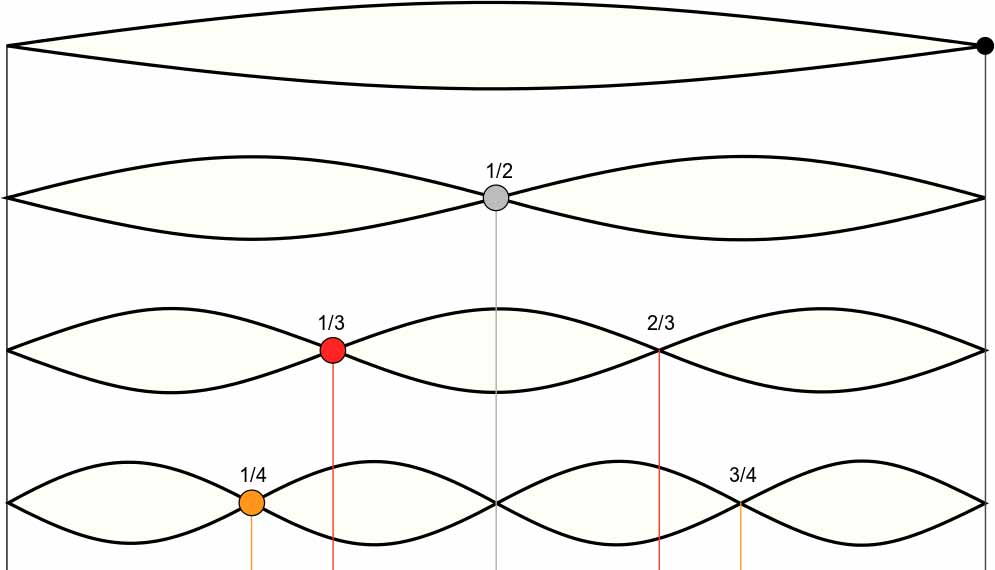The whole tone (1:1), octave (1:2), fifth (2:3) and fourth (3:4).

## Polygons:

 Polygon Sum of Angles Hertz Musical Note Circle 360° 360 Hz F# (perfect octave up from triangle) Square 360° 360 Hz F# (perfect octave up from triangle) Triangle 180° 180 Hz F# (one octave down) Pentagon 540° 540 Hz C# (harmonic fifth of square and triangle) Hexagon 720° 720 Hz F# Heptagon 900° 900 Hz A# (completes an F# major chord in perfect harmony) Octagon 1080° 1080 Hz C#

Geometry is expressed by tones.

The Platonic solids also produce a perfect 3 part major chord in the key of F#.

## Platonic Solids:

 Polyhedra Sum of Angles Hertz Musical Note Tetrahedron 720° 720 Hz F# Cube 2160° 2160 Hz C# Octahedron 1440° 1440 Hz F# Icosahedron 3600° 3600 Hz A# (completes another F# major chord)

# Regular Polygons & Angles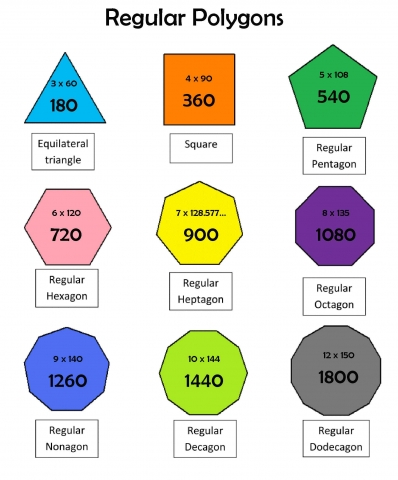Shape Sides Sum of Interior Angles Each Angle Triangle 3 180 60 Square 4 360 90 Pentagon 5 540 108 Hexagon 6 720 120 Heptagon 7 900 128.57… Octagon 8 1080 135 Nonagon 9 1260 140 Decagon 10 1440 144 Sum Totals 6480

# Platonic Solids & AnglesPlato’s Element Volume Shape of Face Faces Edges Corners Sum of Angles Dual “Heaven” or Aether Dodecahedron pentagon 12 30 20 6480 Icosahedron Fire Tetrahedron triangle 4 6 4 720 Tetrahedron Air Octahedron triangle 8 12 6 1440 Cube Water Icosahedron triangle 20 30 12 3600 Dodecahedron Earth Cube square 6 12 8 2160 Octahedron Sum Totals 50 90 50 14400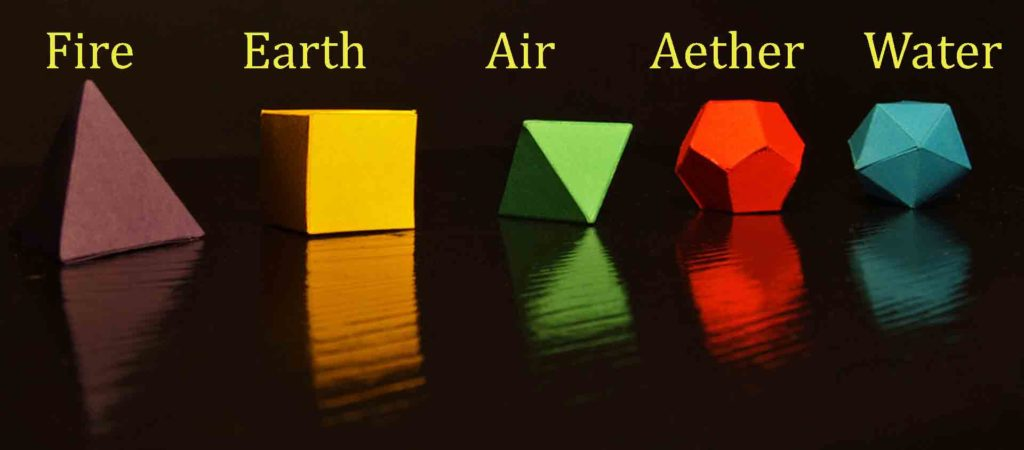# Euler’s Law

Euler’s law states that the number of vertices (corners) plus the number of faces is always equal to the number of edges plus two.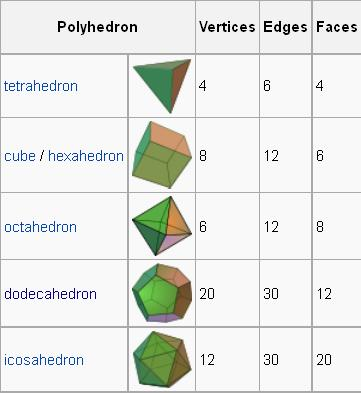For example:

Tetrahedron (4 vertices + 4 faces) = 8

6 Edges + 2 = 8

Octahedron (6 vertices + 8 faces) = 14

12 Edges + 2 = 14

Icosahedron (12 V + 20 F) = 32

30 Edges + 2 = 32

Cube (8 V + 6 F) = 14

12 Edges + 2 = 14

Dodecahedron (20 V + 12 F) = 32

30 Edges + 2 = 32

In another way: V + F = E + 2

This ‘2’ occurs in the equation to represent the ‘poles of spinnability’ according to Buckminster Fuller.

“All systems, Fuller explains, can be spun about a central axis. An axis has two poles (e.g., north and south), and thus at any given time, two vertices must be poles. Subtract the two poles from the total number of vertices to get a number exactly equal to the combined number of edges and faces.”4

Angle Deficiency – the angle left as a gap when a polyhedron’s vertex is folded flat.

Rene Descartes discovered that the sum of a convex polyhedron’s angle deficiencies always equals 720 degrees.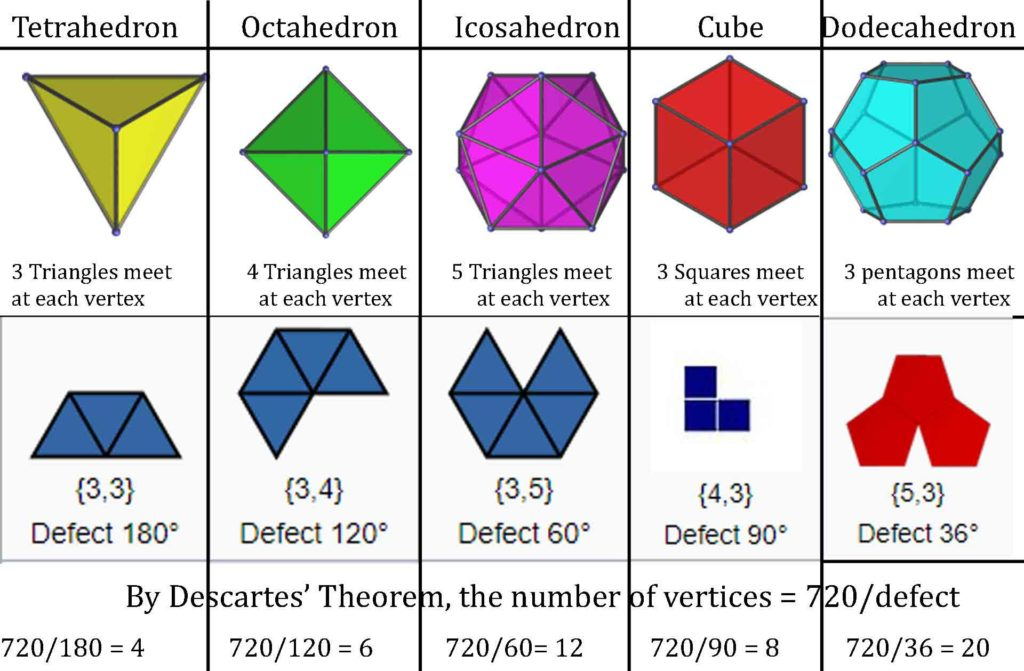1. Critchlow, Keith, Time Stands Still, Brecourt Academic, 2nd edition, 2007
2. ibid.
3. Schneider, Michael, A Beginner’s Guide to Constructing the Universe, Harper Perennial, 1994
4. Edmondson, Amy, A Fuller Explanation: The Synergetic Geometry of R. Buckminster Fuller, Burkhauser Boston, 1987